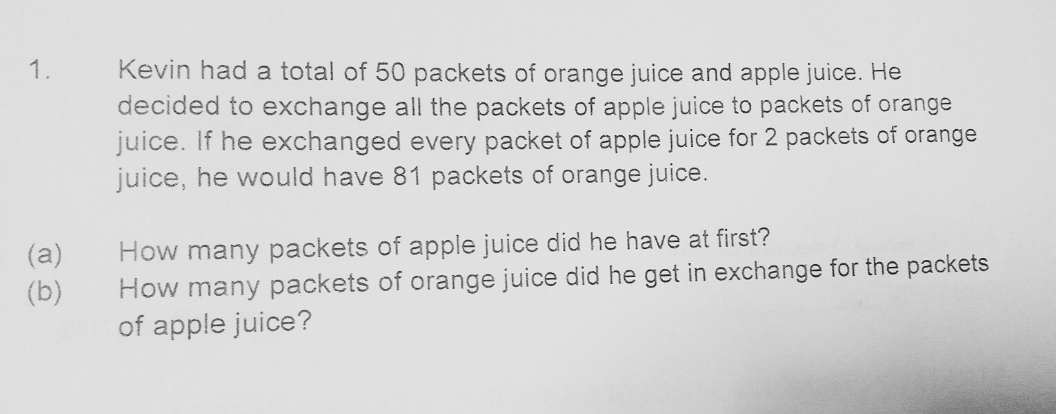# QuestionHi appreciate help on this question. Thks

Each exchange will increase the total by 1

50 – 1 (apple) + 2 (orange) = 51

51 – 1 (apple) + 2 (orange) = 52

….and so on

From 50 to 81, an increment of 81 – 50 = 31

(a) Apple juice at first -> 31

(b) Orange juice from exchange -> 31 × 2 = 62

0 Replies 1 Like ✔Accepted Answer

(a)
81 – 50 = 31

(b)
31 x 2 = 62

Ans : (a) 31 packets of apple juice; (b) 62 packets of orange juice.

0 Replies 1 Like